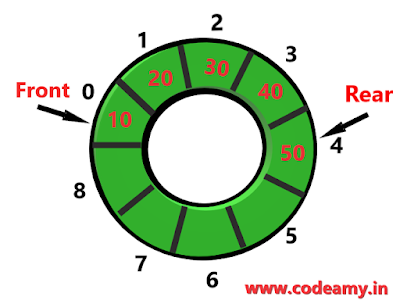# Everything You Need To Know About Circular Queue - C & Algorithm

In this tutorial, you learn about the c circular queue in data structure. In this, we cover the circular queue algorithm - insertion and deletion and implements the algorithm in the running program with the c program.

Let understand about circular queue and advantage over linear queue or simple queue. To learn about the Queue data structure and Queue program in C.

A circular queue is a linear data structure that is implemented in circular form rather than a straight array and avoids the wastage of space in queue using arrays. If we joint both ends of the linear queue then it overcomes the problem of unutilized space in linear queue implemented as an array this is the main advantage of the c circular queue.Circular Queue

## Circular Queue Algorithm

Two operations of the circular queue - Insertion, and Deletion. We use an array of data structures to stored elements.Circular Queue Algorithm

Insertion -  The first element insertion takes place in the circular queue then you have to increase your front and rear from "front=-1" and "rear=-1" then you have to shift both with an increment of +1 and your front and rear at "front=0" and "rear=0" then you insert your first element otherwise your "front=0" is fixed and increment your "rear=rear+1" to insert more.
``````
Start
If ((rear = = size-1 && front==0) || (rear = = front - 1))
printf("Circular Queue is Overflow");
Else If (rear = = size -1 && front ! = 0)
rear=0;
CQ[rear]=item;
Else If (front = = -1)
front=0;
rear=0;
Q[rear]=item;
End
``````
Deletion - If "front==-1" than deletion is not possible then check front and rear both are at the same place then a front and rear both shifted to "front=-1" and "rear=-1" otherwise "front==size-1"(where size is the size of queue whose both ends are joint) then shift "front=0" or at last you increase your front with "front=front+1" to delete.

``````
Start
If (front = = -1)
printf("Circular Queue is Underflow");
Else
item=CQ[front];
If (front = = rear)
front = -1;
rear = -1;
Else If (front = = size - 1)
front=0;
Else
front = front +1;
printf("%d is delete");
End
``````
I think you understand the circular queue algorithm, if not please revisit the above paragraph because below I implement the algorithm in the c program.

## Menu driven program for circular queue in C

``````
/* write a menu driven program using c circular queue.
the menu includes Insert, delete, display, exit
*/
#include<stdio.h>

#define size 5

void cinsert();
void cdelete();
void cdisplay();
int cqueue[size];
int rear = - 1;
int front = - 1;

int main()
{
int choice;
while (1)
{
printf("\n 1.Insert element to CIRCULAR QUEUE \n");
printf("2.Delete element from CIRCULAR QUEUE \n");
printf("3.Display all elements of CIRCULAR QUEUE \n");
printf("4.Quit \n");
printf("Enter your choice : ");
scanf("%d", &choice);
switch (choice)
{
case 1:cinsert();
break;
case 2:cdelete();
break;
case 3:cdisplay();
break;
case 4://exit(0);
default:printf("Wrong choice \n");
}
}
}

void cinsert()
{
int item;
printf("Insert the element in queue : ");
scanf("%d", &item);
if ((rear == size - 1 && front == 0) ||(rear == front-1))
{
printf("Circular Queue Overflow \n");
}
else
{
if (rear== size -1 && front != 0)
{
rear = 0;
cqueue[rear]=item;
}
else
{
if(front==-1)
{
front=0;
rear = 0;
cqueue[rear] = item;
}
}
}
}

void cdelete()
{
int item;
if (front == - 1)
{
printf("Circular Queue Underflow \n");
}
else
{
item = cqueue[front];
if(front==rear)
{
front=-1;
rear=-1;
}
else if(front==size-1)
{
front=0;
}
else
{
front=front+1;
}
printf("Element deleted from queue is : %d\n", cqueue[front+1]);
}
}

void cdisplay()
{
int i;
if(front == -1)
printf("\nCircular Queue is Empty!!!\n");
else
{
i = front;
printf("\nCircular Queue Elements are : \n");
if(front <= rear)
{
while(i <= rear)
printf("%d \n",cqueue[i++]);
}
else
{
while(i <= size - 1)
printf("%d \n", cqueue[i++]);
i = 0;
while(i <= rear)
printf("%d\n",cqueue[i++]);
}
}
}
``````
Output

What are the real life examples of the circular queue?  Comment your answer below.

Happy Coding 😊

If you have any doubts, Please let me know